# Modelling audio trilateration

Modelling audio trilateration in Python! Read: if three people hear a sound at different times, can they find the common location?

Trilateration is the act of finding a location given a set of distances from known locations. It is a little different from triangulation where you know the angles from known locations.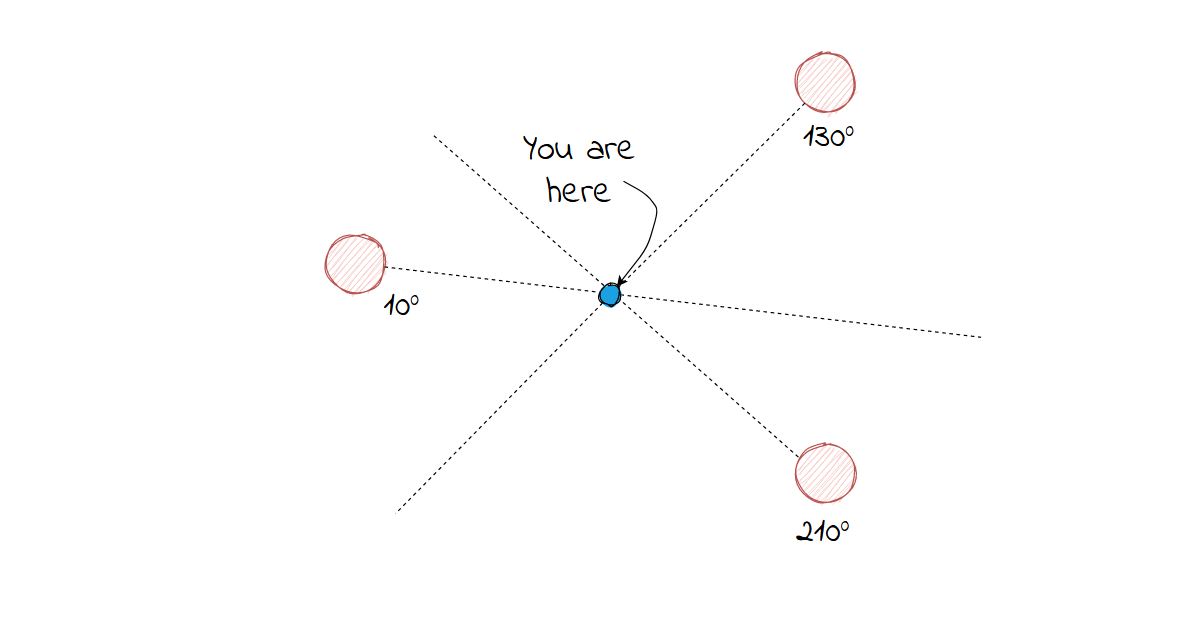Triangulation

With triangulation, you measure the angle and find the intersections.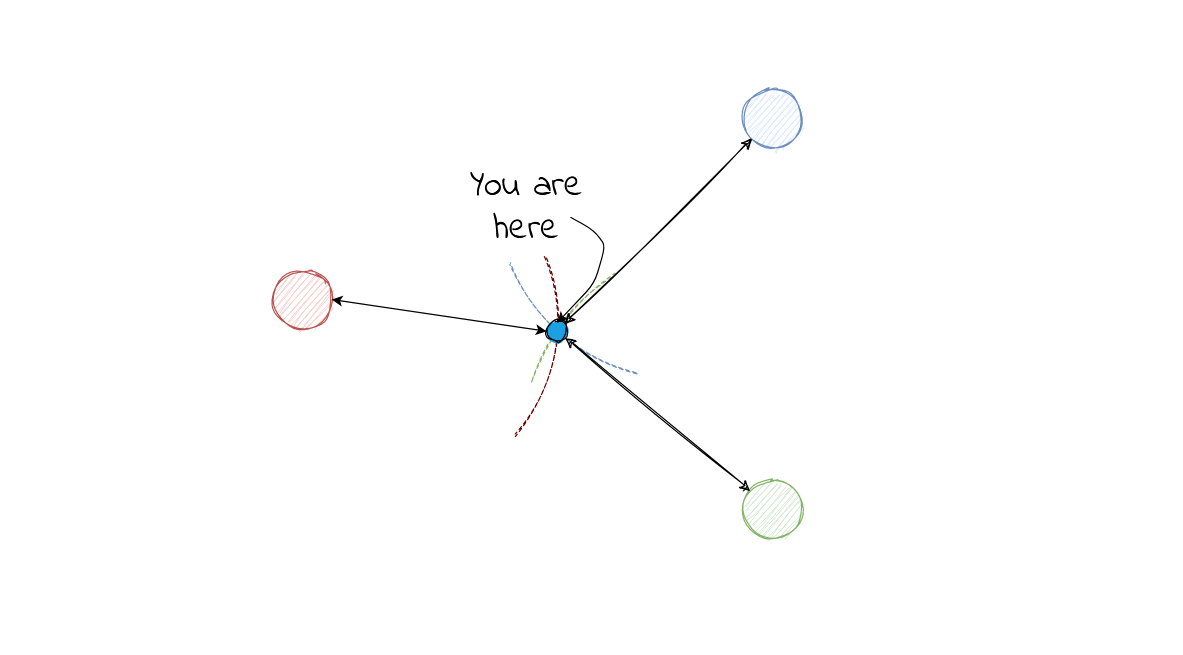Trilateration

With trilateration you find the distance.

Now to picture the problem, since audio signals radiate out from a single point, you don't know the direction, only the time which each node detects the sound.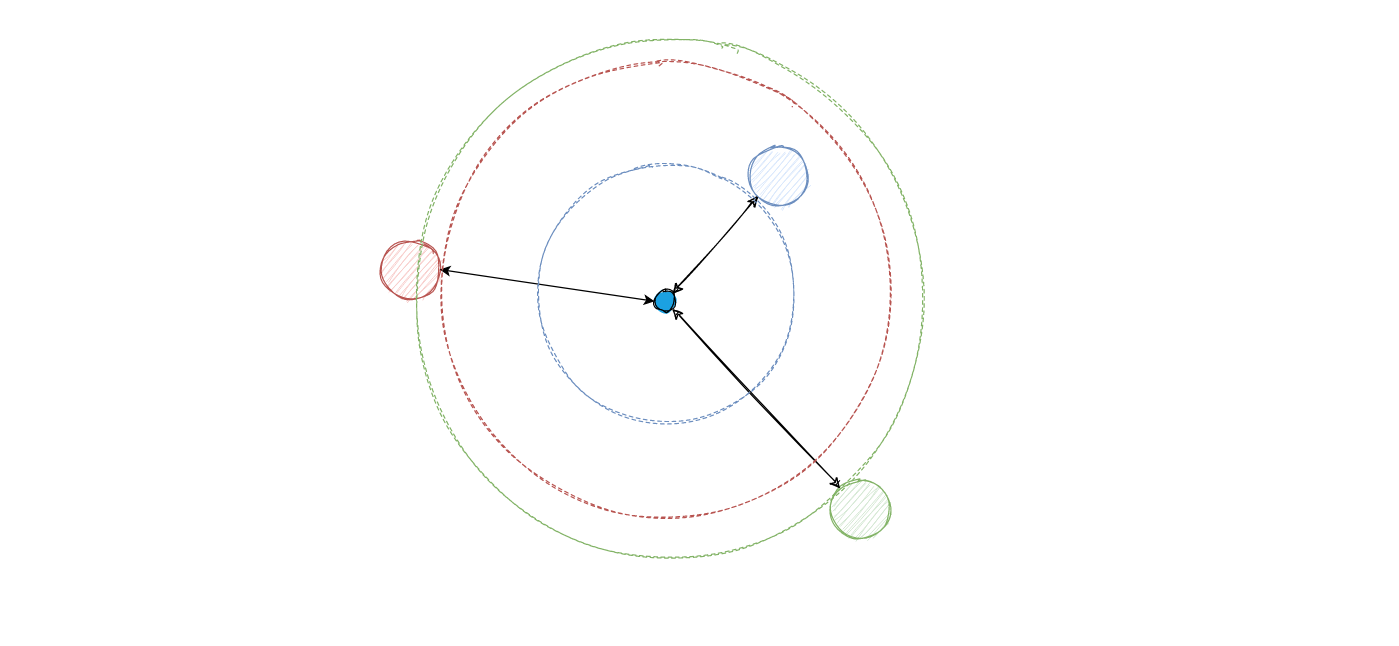Each node detecting the sound

In terms of the an observer timing when each node would detect the sound, the information returned would be in the diagram below.

However, if you flip the perspective and consider it from the point of view from the nodes, this is actually equalvalent to each node emitting a signal at the same time, and this could actually be recording the time the signal of each node reaching the origin of the sound.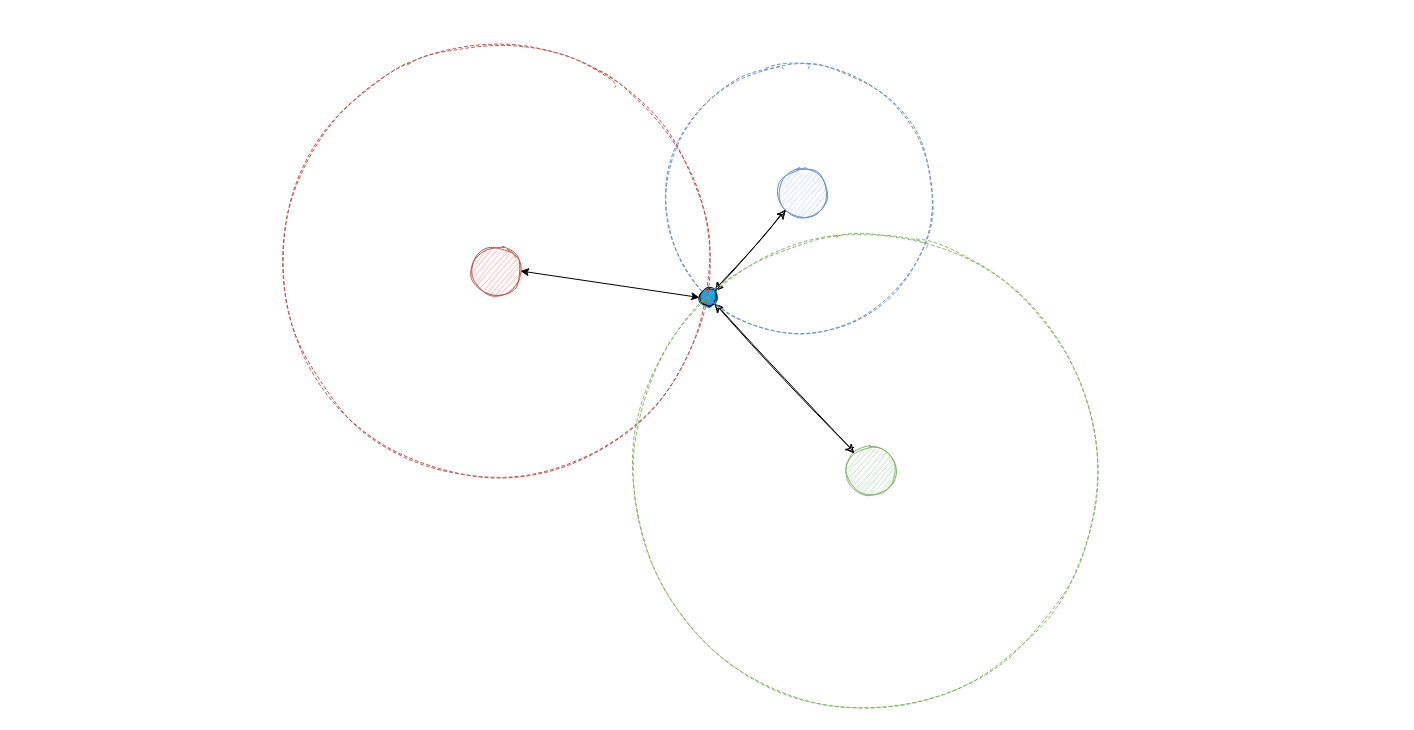Changed reference frames

The point where all these signals intersect is then the origin. Given the location of these nodes, we can simply solve the system of equation of which these three circles intersect, with the radius being given by the time which each signal is received by the node multiplied by the speed:

$(x - x_{\text{node}})^2 + (y - y_{\text{node}})^2 = t_{\text{node}} \times v_{\text{sound}}$

However, this would be considering a perfect system. If there is some level of uncertainty when it comes to detecting the time of the sound, then there wouldn't be a point which three circles intersect.

Consider a listening node detecting a sound at time $t$. The range of possible locations that this sound could have originated from would be a ring around this node. If it were to detect a sound at time $t + \delta t$, then this would be represented as another concentric circle wider than the first. Now given that $\delta t$ is the jitter that a node detects the sound,

$t < t_{\text{actual} < t+\delta t}$

This means that the location of the sound's origin can lie anywhere between these two concetric circles.

Instead of a nice geometrical solution by drawing intersecting circles, now it is not so clear as the location of possible origins is represented as a donut centered at each node.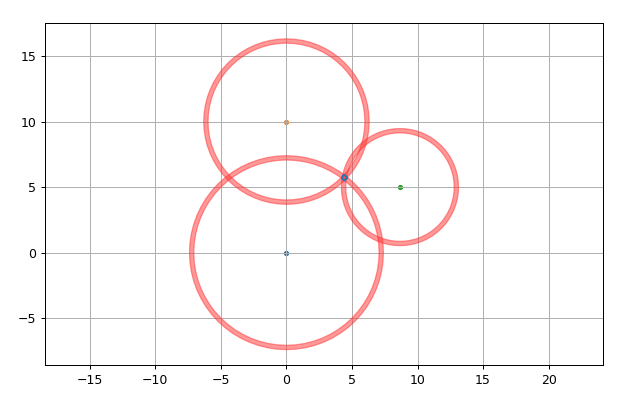Donut possibilities

Therefore, instead of an analytical solution, I resorted to using a numerical one. Since Python has some great GIS libraries, I used the shapes that you can generate with these libraries to help generate a search area:

1. Draw two circles and subtract the smaller circle from the larger one to determine the band of possible origins.
2. Repeat 1. for each node.
3. With these three bands, obtain the shape by performing an intersect operation on each of the bands, and then calculate the area.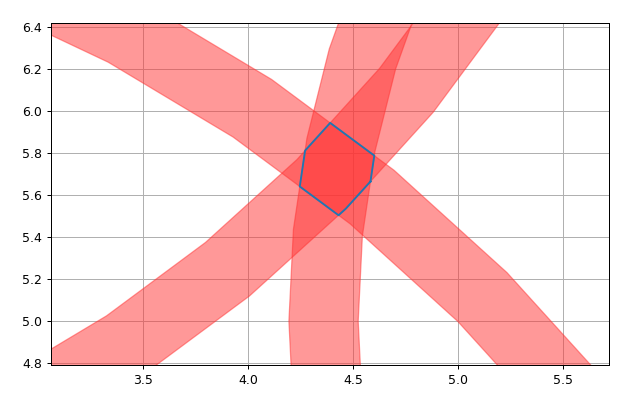Example area to search

As seen, the shape is a little irregular, so it is not very practical to describe as an empirical solution.

The next step I did was to plot it in Matplotlib to determine graphically if the answer made sense (it did), and to provide a visualization of how it would look like. I also added the option to manually click the diagram to generate the origin, and to visualize what the search area would look like. It works even for origins outside of the listening nodes!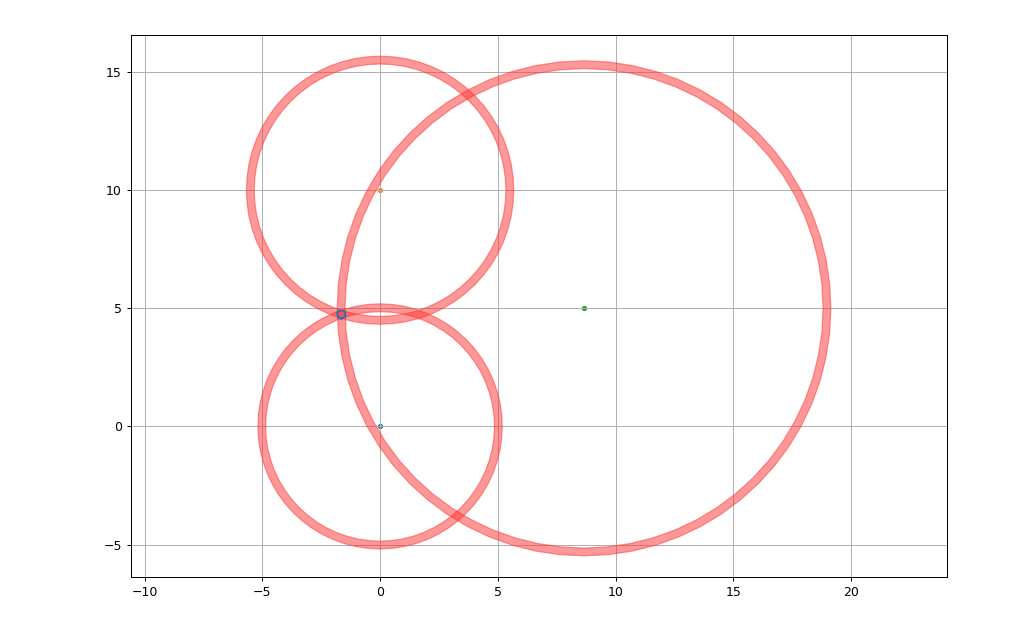Origin outside of listening nodes

This was a simple exploration of how audio trilateration with some error can be modelled in Python, I thought it was fun to build, and hopefully it will be useful to someone out there!